Most Affordable JEE | NEET | 8,9,10 Preparation by Kota's Top IITian Doctor Faculties

# Circular Motion Practice Problems with Answers Physics Class 11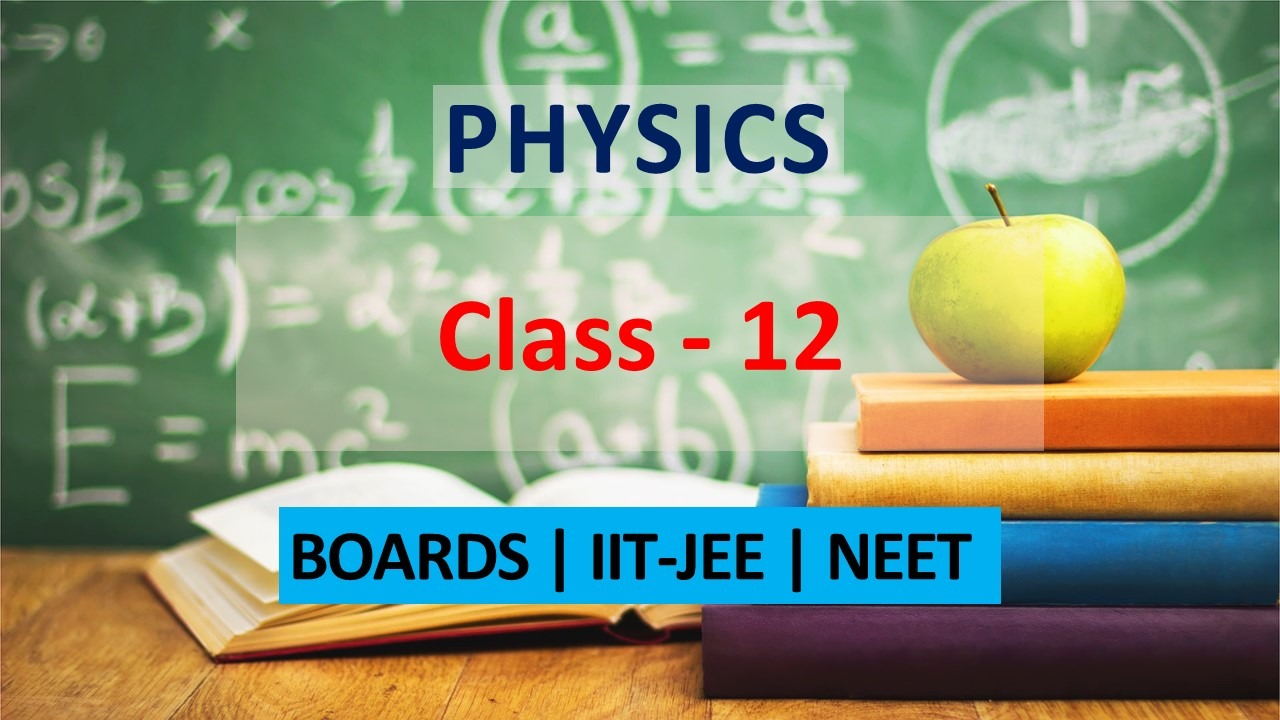`
Get circular motion practice problems with answers for class 11 physics. View 11th Physics important questions for exam point of view. These important questions will play significant role in clearing concepts of Physics. This question bank is designed by expert faculties keeping NCERT in mind and the questions are updated with respect to upcoming Board exams. You will get here all the important questions for class 11 Physics chapters. Improve your concepts of circular motion with these practice problems with answers and increase your marks in exams. Click Here for Detailed Notes of Circular Motion along with other chapters.
Q. Can a particle accelerate when its speed is constant? Explain.
Ans. A particle can be accelerated if its velocitychanges. A particle having uniform circular moton has constant speed but its dirction of motion changes continuously. Due to this, there is change in velocity and hence the particle is accelerated. Is it possible for a body to have constant speed but variable velocity? If so, give example.

Q. Is it possible for a body to have constant speed but variable velocity $?$ If so, give example.
Ans. Hes. When a body moves in a circle with constant speed, its velocity is variable. This because velocity is a vector quantity whose magnitude is speed but its direction changes continuously due to the change of the direction of motion.

Q. Can a body have a constant velocity and still a variable speed?
Ans. No. Velocity is a vector quantity and speed is its magnitude. A vector quantity changes if either its magnitude or direction changes. Since the magnitude of velocity (i.e., speed) changes and hence the body can not have a constant velocity.

Q. Is circular motion possible at constant speed or at constant velocity? Explain.
Ans. Circular motion is possible at constant speed because in circular motion, the magnitude of the velocity i.e., speed remains constant while the direction of motion changes continuously.

Q. Is uniform circular motion an example of uniform acceleration. Why?
Ans. No, since acceleration is always towards the centre.

Q. Name the physical quantity which remains same in an uniform circular motion.
Ans. Kinetic energy and speed.

Q. Two particles are moving with common speed v such that they are always at a constant distance ' d ' apart and their velocities are always equal and opposite. After what time, they turn to their initial positions?
Ans. The two particles should be moving in circular path of radius d / 2 with same speed. Since tangent at any point gives the direction of velocity, they will always be opposite. They will turn to their initial position after completing one circular path.

Q. Why do the passengers of a car rounding a curve are thrown outward?
Ans. When the car turns round a curve, the passengers sitting in the car experience an outward force, i.e. centrifugal force due to the absence of the necessary centripetal force.

Q. Is it possible to be accelerate a particle if it is travelling at constant speed?
Ans. Yes, when a particle moves in a circles with constant speed, its velocity is variable because the direction of motion is continuously changing. accelerating.

Q. What do you mean by centrifuge? Explain its principle.
Ans. It is a device used for separating mixtures of substances with different densities. It works on the principle that the centrifugal force, $F=m r w^{2}$ acting on a body in circular motion is, (i) Directly proportional to mass ( $m$ ) of the body. (ii) Directly proportional to the distance of the body from its axis of rotation. The heavier particles experience large amounts of centrifugal force, i.e., they will be push outward, and get separated from the lighter particles.

Q. Moon is continuously revolving round the earth without falling towards it. Justify, why it doses so?
Ans. For revolving round the earth, the moon provides itself the necessary centripetal force at the expense of its weight. We know that a body falls under the effect of its weight. Since the weight of the moon gets used in providing it the necessary centripetal force, it revolves round the earth, without falling towards it.

Q. For uniform circular motion, does the direction of the centripetal force depend upon the sense of rotation?
Ans. The direction of the centripetal force does not depend, whether the body is moving in clockwise or anticlockwise direction. It is always directed along the radius and towards the centre of the circle.

Q. Why does a cyclist lean to one side, while going along a curve? In which direction does he lean? Or Why does a cyclist lean inward while negotiating a curve? Explain with a diagram.
Ans. While going along a curve, the cyclist leans to one side, so as to provide himself the necessary centripetal force. He leans in the inward direction i.e., towards the centre of the circular curve.

Q. A body is suspended by a string of length and is projected horizontally with velocity . Calculate the tangential and radial acceleration when the string rises by from its initial position.Also find the difference in velocity.
Ans. $l=1 m,$ applying conservation of energy, we have $m g(1-\cos 60)=\frac{1}{2} m v_{B}^{2}$ $V_{B}=2 g\left(1-\frac{1}{2}\right)=g$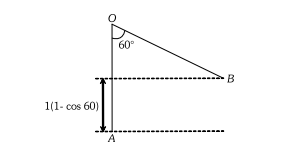Centripetal acceleration $=g \sin 60^{\circ}=\frac{\sqrt{3}}{2} g$ Velocity difference $=v_{B}-v_{A}=10-4 m s^{-1}$
Q. The acceleration associated with a mass moving in a circular path is to be found. It is given that the velocity at any instant is where is a constant. Classify the motion and find acceleration.
Ans. since velocity changes with time, the motion in circular path involves tangential acceleration. So it is a non-uniform circular motion. Net acceleration $=a_{n} \sqrt{a_{r}^{2}+a_{t}^{2}}=\sqrt{\left(K^{2} r t^{2}\right)^{2}+(K r)^{2}}=K r \sqrt{1+K^{2} t^{4}}$
Q. What is the need for banking the tracks?
Ans. On a plane road, the centripetal force required to negotiate the curve is provided by the force of friction between the tyres of the vehicle and the surface of the road. Thus, the force of friction depends upon the nature of the surfaces. The force of friction may provide the necessary centripetal force if the speed of the vehicle is small enough. On the other hand, if the speed of the vehicle at the curve is not small enough, then theforce of friction will be unable to provide the necessary centripetal force to the vehicle and it may skid away. Therefore, to provide the necessary centripetal force to the vehicle at higher speeds, roads are banked.
Q. A train round an unbanked circular track of radius at a speed of . The mass of the train is. What provides the centripetal force required for this purpose ? The engine or the rails ? The outer or the inner rail? Which rail will wear out faster, the outer or the inner rail? What is the angle of banking required to prevent wearing out of the rails?
Ans. Given that and The centripetal force required for negotiating the curve on the unbanked road is provided by the lateral thrust by the outer rail on the flanges of the wheels. According to Newton's third law of motion the train exerts an equal an opposite force on the outer rail. Hence, the outer rail will wear out faster than the inner rail.Further, given that,Speed of train, Radius of the bend, . If is the angle of banking required to prevent wearing out of the rails,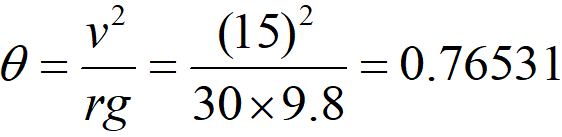$\theta=37^{\circ} 25^{\prime}$
Q. A large mass and a small mass hung at the two ends of a string that passes over a smooth tube. The mass moves around in a circular path which lies in the horizontal plane. The length of the string from the mass to the top of the tube is and is the angle this length makes with the vertical. What should be the frequency of rotation of the mass so that mass remains stationary?
Ans. The various forces acting on $M$ and $m$ are $T=M g$ $T \cos \theta=m g$ $\begin{array}{l}{T \sin \theta=\frac{m v^{2}}{r}=m r \omega^{2}} \\ {\text { Where } r \text { is the radius of the circular path and } \omega} \\ {\text { is the angular velocity. }} \\ {r=l \sin \theta} & {\text { [From(iii)] }}\end{array}$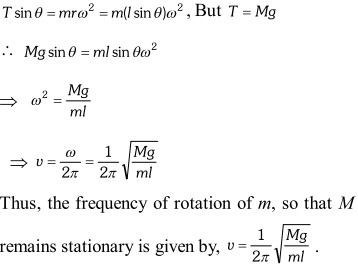Q. A conical pendulum consist of a string P Q whose upper end is fixed and lower and is attached with a bob. The bob is drawn aside, and given a horizontal push so that it describes a horizontal circle with angular speed in such a way that P Q makes a constant angle with the vertical. As the string traces the surface of a cone, it is known as a conical pendulum. Deduce an expression for the time period for the pendulum.
Ans.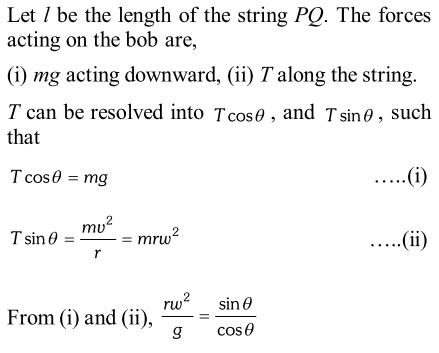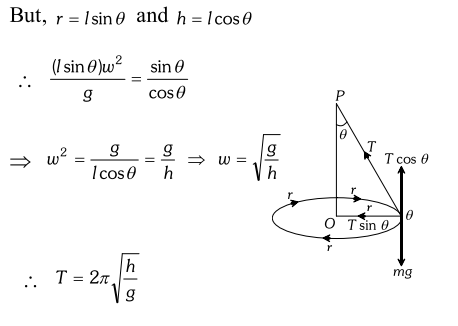Q. A cyclist moving with a velocity of approaches a -turn of radius his speed at a rate of . Calculate the acceleration of the cyclist on the turn.
Ans. $v=7.5 \mathrm{ms}^{-1}, r=80 \mathrm{m}$ centripetal acceleration is,When the cyclist applies brakes at $P$ of the circular turn, the cyclist applies brakes at $P$ of the circular Acceleration will act opposite to the velocity i.e., $a_{t}=0.5 \mathrm{ms}^{-2}$ $a=\sqrt{a_{c}^{2}+a_{t}^{2}}=\sqrt{(0.7)^{2}+(0.5)^{2}}=0.86 \mathrm{ms}^{-2}$ Let $\theta$ be the angle made by net acceleration with the velocity of the cyclist, then $\tan \theta=\frac{a_{c}}{a_{t}}=\frac{0.7}{0.5}=1.4$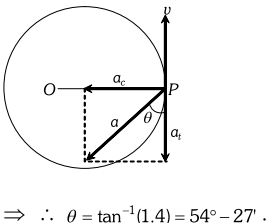Q. A car of mass moves with a constant speed over a (a) horizontal flat surface (b) a convex bridge (c) a concave bridge. What force is exerted by the car on the bridge in each of these cases, as it passes the middle point of the bridge ? Take radius of curvature of the bridge in the last cases as r.
Ans. The car is acted upon in the Vertical  direction by two forces: its weight $M g$ and the normal reaction $R$. (a) When the car runs over a horizontal bridge, the normal reaction, say $R_{1}$ is just equal and opposite to its weight $M g$ i.e., $R_{1}=M g$ Thus, force on the bridge is equal to the weight of the car. (b) When the car runs over a convex bridge, it requires a centripetal force vertically downwards. If $R_{2}$ is normal reaction, then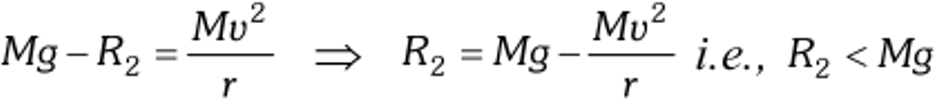Thus, force on the bridge is less then the weight of the car.
Q. A pendulum consisting of a bob of mass and string of length is moved upto the horizontal position and released. What should the minimum strength of the string be to withstand the tension as the pendulum passes through the position of equilibrium?
Ans. When the bob is released from the horizontal position. A, it will reach the equilibrium position by moving along circular path as shown figure. At point , the bob of the pendulum is acted upon by two forces: its weight m g and tension in the string. The resultant of these forces provides the necessary centripetal force i.e.,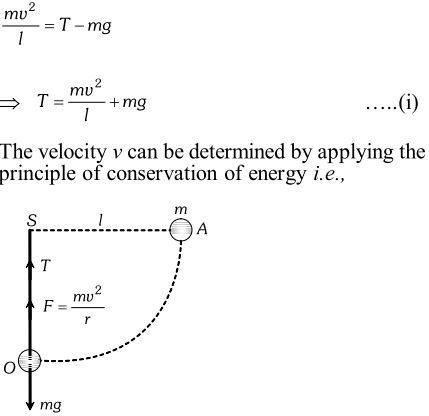Kinetic energy at of the bob point $O=$ Potential energy at point $A$ $\frac{1}{2} m v^{2}=m g l \Rightarrow v=\sqrt{2 g l}$ Substituting for $v$ in equation (i), we have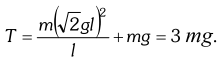The string should have minimum strength to stand a tension three times the weight of the bob.
eSaral provides you complete edge to prepare for Board and Competitive Exams like JEE, NEET, BITSAT, etc. We have transformed classroom in such a way that a student can study anytime anywhere. With the help of AI we have made the learning Personalized, adaptive and accessible for each and every one. Visit eSaral Website to download or view free study material for JEE & NEET. Also get to know about the strategies to Crack Exam in limited time period.

Kazija Ali
April 10, 2023, 6:35 a.m.
I don't undestand
Kazija Ali
April 10, 2023, 6:35 a.m.
I don't undestand
Kanika
Sept. 16, 2022, 7:18 a.m.
if you post some numericals then it will be better than these theory questions
Kazija Ali
April 10, 2023, 6:35 a.m.
True
Bhakti
March 13, 2021, 5:48 a.m.
Plz post the numerical plz
Aarya
Sept. 21, 2020, 7:22 p.m.
Thank u for the questions provided...it was very helpful for revising our theory
Zaqi
Sept. 28, 2023, 6:35 a.m.
Yes....👍❤️
Zaqi
Sept. 28, 2023, 6:35 a.m.
Yes....👍❤️
vyshnavi iit jee aspirant in 2022
Aug. 13, 2020, 8:35 p.m.
i suggest you to post some numericals instead of this hand written questions so that it will be helpful for students preparing for iit jee or neet exam